## 1. Overview

Fourier Analysis has taken the heed of most researchers in the last two centuries. One can argue that Fourier Transform shows up in more applications than Joseph Fourier would have imagined himself!

In this tutorial, we explain the internals of the Fourier Transform algorithm and its rapid computation using Fast Fourier Transform (FFT):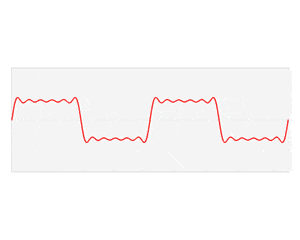We discuss the intuition behind both and present two real-world use cases showing its importance.

## 2. Introduction

Expanding a Function into a summation of simpler constituent Functions has redirected several scientists to tune into understanding numerous fields, e.g., overtones, wireless frequencies, harmonics, beats, and band filters. When Fourier first introduced his analysis and the possibility of expressing a periodic function (e.g.,) using a sum of periodic functions, he ran into multiple criticisms.

For example, Laplace (who was “the” physicist of his time) could not believe that aseries could express a. In 1811, Fourier resubmitted his work with an important addition, the Fourier Transform, which showed that not exclusively it is possible to expand Periodic Functions but non-Periodic ones too.

### 2.1. Computation of Fourier Transform

The study of Linear Systems and Signal Processing recognized the importance of Fourier Transforms. However, it did not reflect on numerical computation because of the number of arithmetic operations needed to calculate the discrete version of the Fourier Transform. At least that was the case until James Cooley and John Tukey introduced the Fast Fourier Transform algorithm.

## 3. Discrete Fourier Transform (DFT)

The Discrete Fourier Transform ofpoints is given by:Where### 3.1. De Moivre’s Formulaand its powers construct the Fourier Transform. Althoughhas two forms, using the exponential one () should serve us better in understanding its behavior.

Let;will be the first root.

The figure below shows the eight different roots whereis the first blue line, making a 45 degree (), and each of the following blue lines (also where the annotations are) will be theroot offor: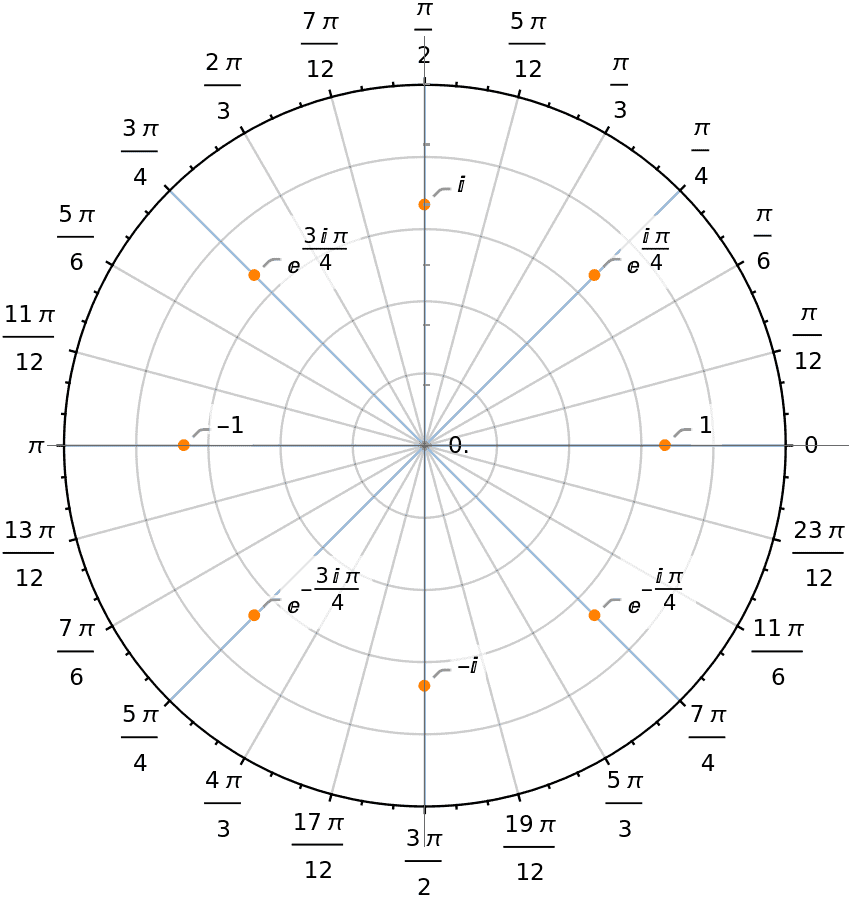We can think of the multiplication between the different powers ofand the input sequence as asking eachto capture the frequency it senses from the current input sequence. When the sum is infinite (i.e., having too many‘s sensing the frequencies), it converges to the exact input sequence: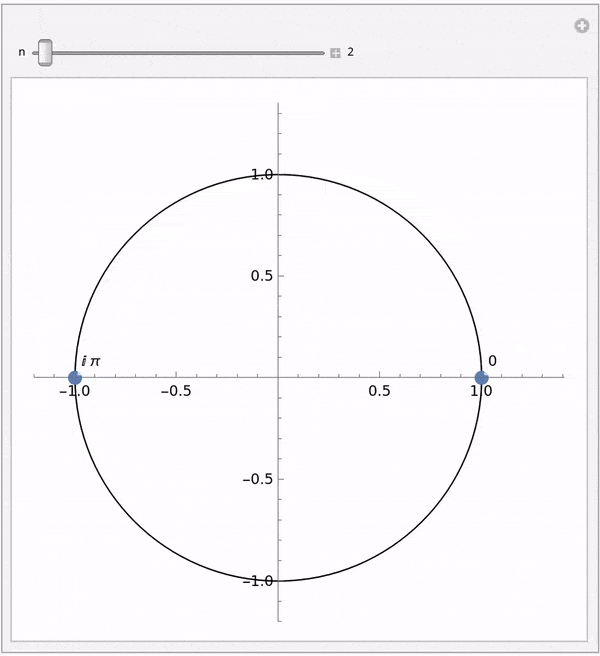Each computation ofrequires(complex) multiplication and(complex) additions. Hence, the complexity of total computation is(complex) multiplications and(complex) additions, complexity of.

## 4. Fast Fourier Transform

The observation made by Cooley and Tukey was that ifis a composite number, a reduction in complexity exists. We can notice that as we square, we get.

For example,is.for: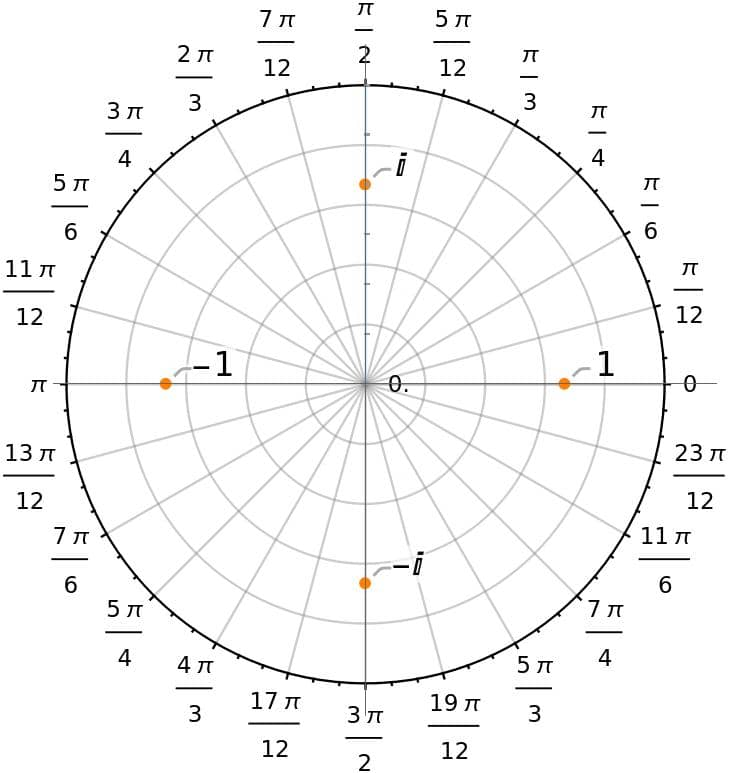### 4.1. DFT Vector Notation

To start our optimization attempt, it would be easier to work with and understand the vector notation of DFT:WhereNowis a mesmerizing matrix; we construct it, multiply it by a sequence, and get the Fourier Transform of that sequence. But, we want to multiplybyas quickly as possible.

Normally, such multiplication would take, and the optimization is possible if the matrix contained zeros, but the Fourier matrix has no zeros!

However, it is possible to make use of the special patternwe previously learned so thatcan be factored in a way that produces many zeros (a.k.a FFT). The key idea is to connectto.

For instance, let; we would like to find the connection between### 4.2. Complexity Reduction

So, we are somehow trying to reduce the number of operations by half, but these two matrices are not equal, yet. How about we add a permutation matrix that splits the input sequence into odd and even coordinates?At this point, we succeeded in producing a lot of zeros, but the factorized version seems far from being equal to the original. However, we are pretty close, only if we can find a “fix-up matrix” to correct the previous output.

### 4.3. Introducing a “Fix up Matrix”

Let’s consider the following matrix:The new “fix up matrix” works on the reassembly of the half-sizesmatrices. The form of that matrix is:Whereis a diagonal matrix with entriesandis the identity matrix:The full form would be:What comes next? We reducedto. Keep going to, …,. That’s recursion! There arelevels, going fromdown to. Each level hasmultiplications from the diagonal‘s, to reassemble the half-size outputs from the lower levels. This yields the final count., which is.

Note: In caseis not a power of, we can pad the input sequence with‘s.

For, it would takemultiplications, where FFT would performmultiplications only.

## 5. Applications

Fourier Transform has an indispensable number of applications to be listed. We’ll cast a shadow on Acoustics and Convolution Theorem.

### 5.1. Acoustics

How can Shazam recognize a song/music in a matter of seconds? More surprisingly, it does so regardless it is listening to an intro, verse, or chorus: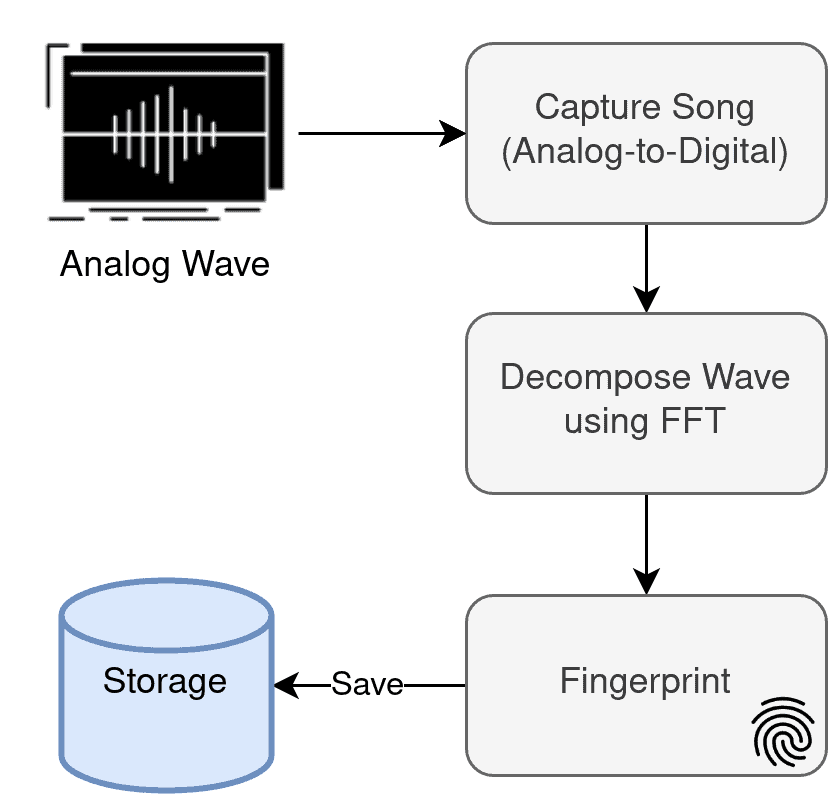There are (roughly) four steps it performs:

• Captures the song using a mobile phone (i.e., analog-to-digital wave conversion)
• Transform the captured function (or wave) into a frequency domain using FFT
• Fingerprint the expanded function
• Compare the fingerprint against the songs fingerprints database

### 5.2. Convolution Theorem

The theorem states that circular convolutions (i.e., periodic convolutions between two periodic functions having the same period) in the spatial domain are equivalent to point-wise products in the Fourier domain.

Letdenote the Fourier Transform andits inverse, we can compute convolutions between functionsandas followsOriginally, the convolution betweenandis calculated withA convolution of sizewith a kernel sizerequiresoperations using the direct method, the complexity would be. On the other hand, FFT-based method would needoperations with complexity: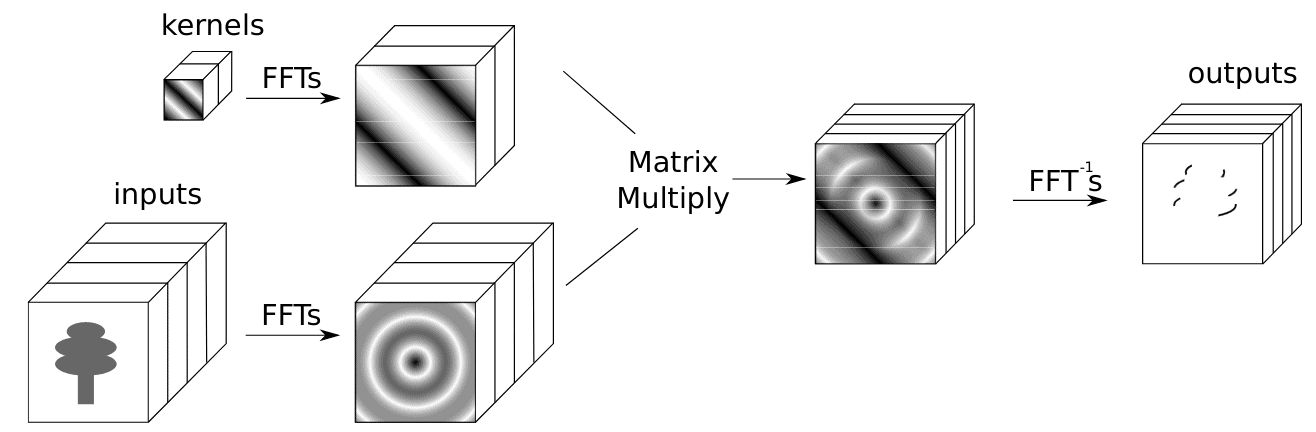The convolution operation is used in a wide variety of applications such as:

## 6. Conclusion

In this tutorial, we have provided a short mathematical analysis of the Fast Fourier Transform algorithm and how it can, surprisingly, impact a lot of fields and applications. The breakthrough of the algorithm only started a few decades ago even though Joseph Fourier introduced his analysis almost two centuries back.